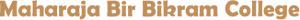Knowledge is the key to immortality Some Programs in C - Part 9 Prepared by Ratul Chakraborty - Associate Professor - Statistics Summary Statistics of ungrouped data The Program ``````#include #include int main() { int i, n; double x, sum = 0, sumsqr = 0, logsum = 0, invsum = 0, variance, SD, CV, AM, GM, HM; printf("Total Observations: "); scanf("%d", &n); for(i=1; i<=n; i++){ printf("\nX%d: ", i); scanf("%lf", &x); sum = sum + x; sumsqr = sumsqr + x*x; logsum = logsum + log(x); invsum = invsum + 1.0/x; } AM = sum/n; GM = exp(logsum/n); HM = n/invsum; variance = sumsqr/n - AM*AM; SD = sqrt(variance); CV = SD/AM*100; printf("\nAM = %g", AM); printf("\nGM = %g", GM); printf("\nHM = %g", HM); printf("\nVariance = %g", variance); printf("\nSD = %g", SD); printf("\nCV = %g", CV); return 0; }`````` Output ``````Total Observations: 5 X1: 24 X2: 56 X3: 17 X4: 28 X5: 10 AM = 27 GM = 22.9721 GM = 19.6803 Variance = 248 SD = 15.748 CV = 58.326`````` Copyright © 2018 - All Rights Reserved - www.mbbcollege.in